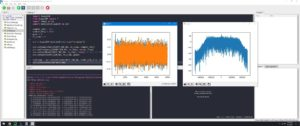We are seeing more and more interest in the Python programming language among the radio community.

With encouragement from Marc Lichtman  (author of a free online textbook ( www.pysdr.org, ), we are keen to help Python lovers to explore what can be done with an RSP.

If you are interested in processing data using the RSP and Python 3 in Windows,  Mike Ladd (mike dot ladd at sdrplay dot com) would love to see what people are doing and how well the set-up works.
Here’s what to do:

Install Python 3 for windows from https://www.python.org

Use the demo script below as a starting point.
————————————————————————–
import SoapySDR
from SoapySDR import * #SOAPY_SDR_ constants
import numpy as np
import matplotlib.pyplot as plt
sample_rate = 1e6
center_freq = 100e6
gain = 0
rx_chan = 0 # only 1 channel on RSP1A
sdr = SoapySDR.Device(dict(driver=”sdrplay”))
sdr.setSampleRate(SOAPY_SDR_RX, rx_chan, sample_rate)
sdr.setFrequency(SOAPY_SDR_RX, rx_chan, center_freq)
sdr.setGainMode(SOAPY_SDR_RX, rx_chan, False) # turn off AGC
sdr.setGain(SOAPY_SDR_RX, rx_chan, gain)
rxStream = sdr.setupStream(SOAPY_SDR_RX, SOAPY_SDR_CF32, [rx_chan])
sdr.activateStream(rxStream) #start streaming
num_samps = 10000
buff = np.array(*num_samps, np.complex64)
print(“samples received:”, sr.ret) # number of samples read or the error code
#shutdown the stream
sdr.deactivateStream(rxStream) #stop streaming
sdr.closeStream(rxStream)
print(buff[0:20])
plt.figure(0)
plt.plot(np.real(buff), ‘.-‘)
plt.plot(np.imag(buff), ‘.-‘)
plt.figure(1)
PSD = 10*np.log10(np.abs(np.fft.fftshift(np.fft.fft(buff)))**2)
f = np.linspace(-sample_rate/2, sample_rate/2, num_samps)
plt.plot(f, PSD)
plt.show()
print(dir(sdr))
print(sdr.listGains(SOAPY_SDR_RX, rx_chan))
print(sdr.getBandwidth(SOAPY_SDR_RX, rx_chan))
print(sdr.listAntennas(SOAPY_SDR_RX, rx_chan))Have fun!
Many thanks to Marc Lichtman for his help.
Marc Lichtman recently presented this interesting video (21 minutes in) on teaching SDR technology to Computer Science students:  https://youtu.be/NALZ-6uMUQA